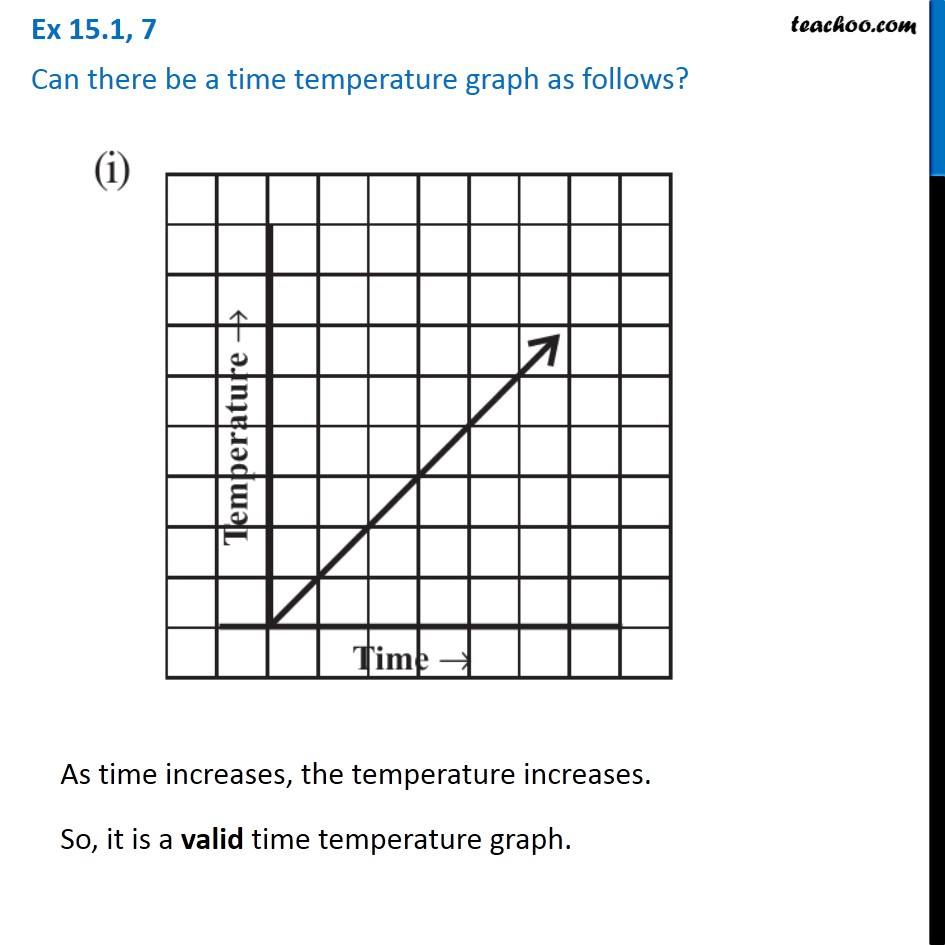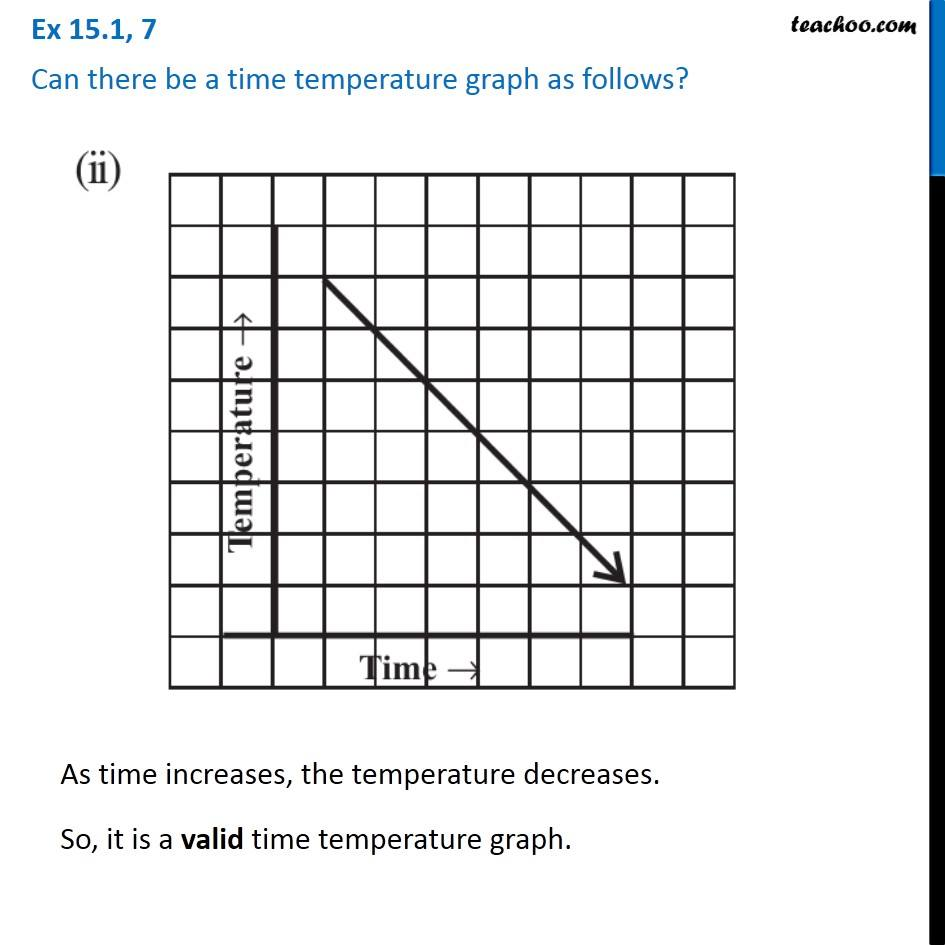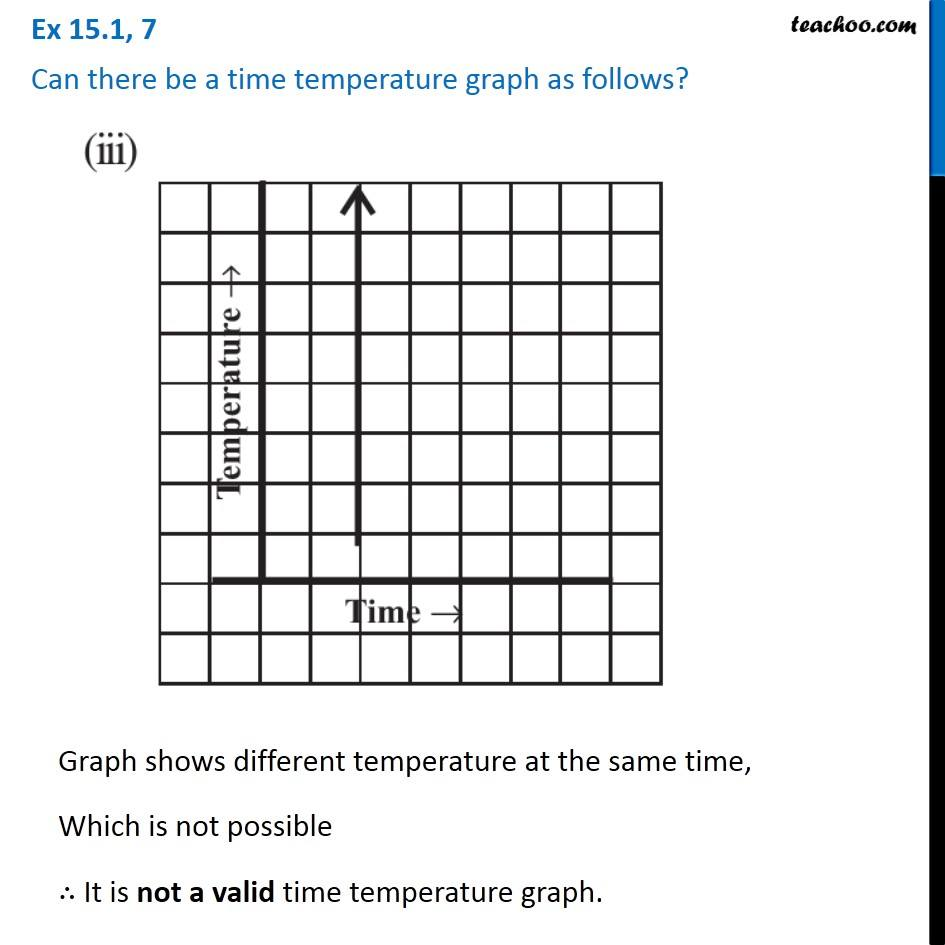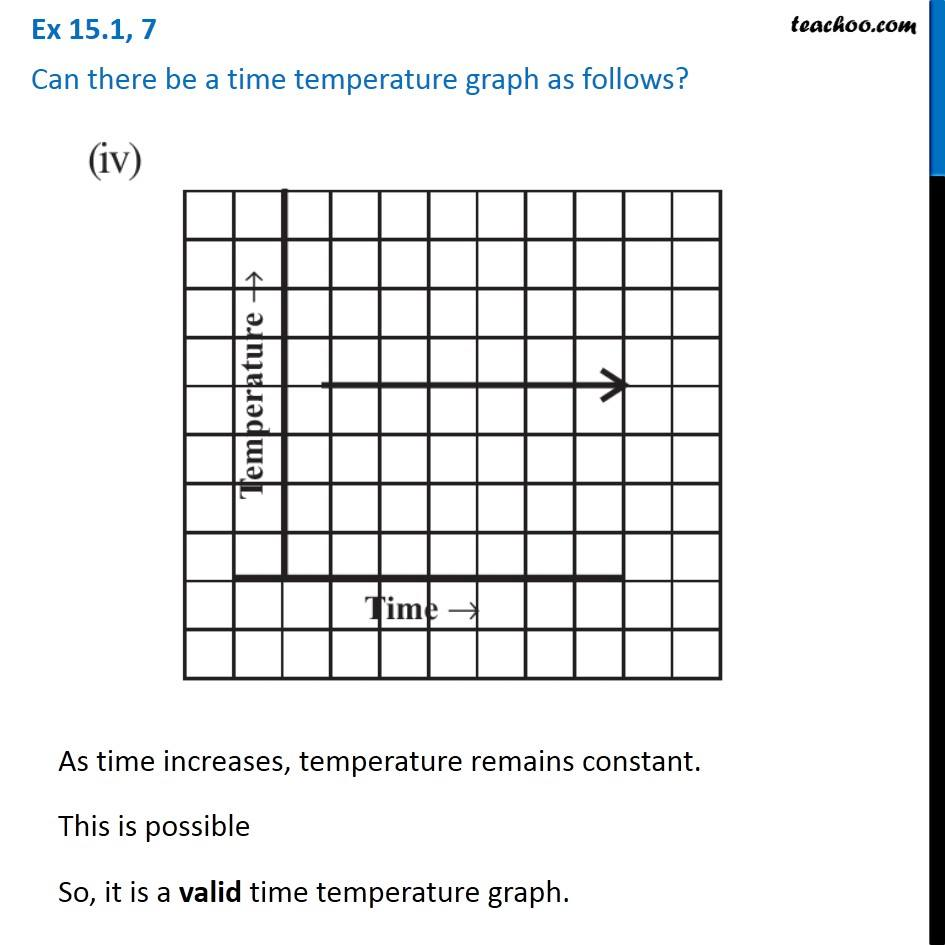Ex 13.1

Chapter 13 Class 8 Introduction to Graphs
Serial order wiseLearn in your speed, with individual attention - Teachoo Maths 1-on-1 Class

### Transcript

Ex 13.1, 7 - Chapter 15 Class 8 Introduction to Graphs - NCERT Can there be a time temperature graph as follows? (i) As time increases, the temperature increases. So, it is a valid time temperature graph (ii) As time increases, the temperature decreases. So, it is a valid time temperature graph. (iii) Graph shows different temperature at the same time, Which is not possible ∴ It is not a valid time temperature graph. (iv) As time increases, temperature remains constant. This is possible So, it is a valid time temperature graph.